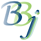# DIM Verb - BBj

For this topic's original documentation, see the DIM Verb.

## Description

DIM X![dimensions] allocates an object array.

DIM N(number) can be used to initialize numeric array elements.

DIM allows more than 3 array dimensions, and the DIMS() function returns information about extra dimensions if used. Note that multi-dimensional arrays use up memory quickly, so this feature should be used sparingly.

BBj does not allow the use of parenthesis to reference or dimension numeric arrays. For example, DIM A(3) generates an !ERROR=20 in BBj whereas BBx converts the line to DIM A.

## Example

 DIM X!; REM ' Create 5-element object array LET X!=123; REM ' number LET X!="hello, world"; REM ' string LET X!=java.lang.Boolean.TRUE; rem ' object LET X!=new java.util.ArrayList(); rem ' object PRINT X![ALL] null 123hello, worldtrue[] LET Y![ALL]=X![ALL]; REM ' performs a shallow copy of the object references in X![]